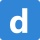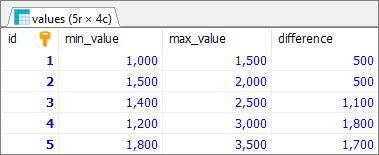Home
IT Knowledge
Inspiration
Languages
EN

# MySQL - get difference between two columns

0 points
Created by:Dirask Community
6390

In this article, we would like to show you how to get the difference between two columns in MySQL.

Quick solution:

``````SELECT `column1`, `column2`, `columnN`,
`column1`-`column2` AS 'alias_name'
FROM `values`;``````

## Practical example

To show how to get the difference between two columns, we will use the following `values` table:MySQL - example data used to get the difference between columns

Note:

At the end of this article you can find database preparation SQL queries.

### Example

In this example, we will get the difference between `max_value` and `min_value` columns from `values` table.

Query:

``````SELECT `id`, `min_value`, `max_value`,
`max_value`-`min_value` AS 'difference'
FROM `values`;``````

Output:MySQL - get the difference between columns - result

## Database preparation

`create_tables.sql` file:

``````CREATE TABLE `values` (
`id` INT(10) UNSIGNED NOT NULL AUTO_INCREMENT,
`min_value` INT,
`max_value` INT,
PRIMARY KEY (`id`)
);``````

`insert_data.sql` file:

``````INSERT INTO `values`
(`min_value`, `max_value`)
values
(1000, 1500),
(1500, 2000),
(1400, 2500),
(1200, 3000),
(1800, 3500);``````

## MySQL - Problems

##### 🚀
Get your tech brand or product in front of software developers.
For more information Contact us

Join